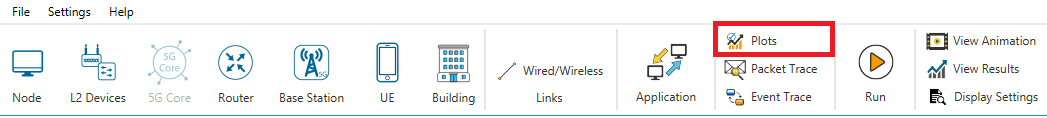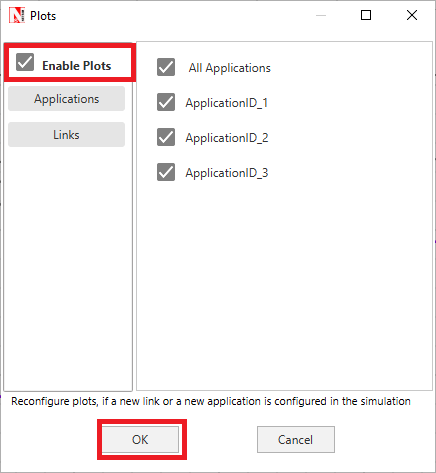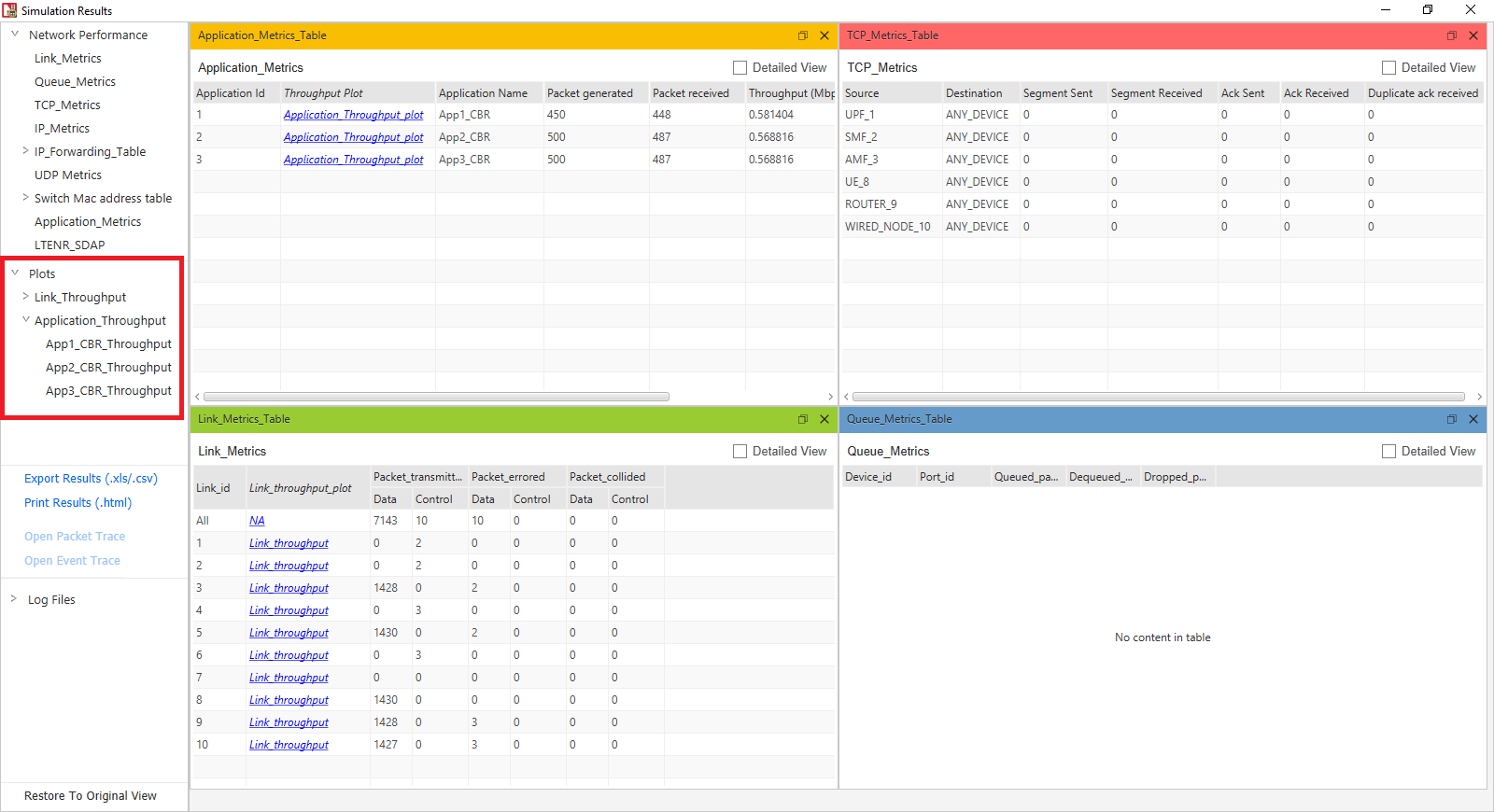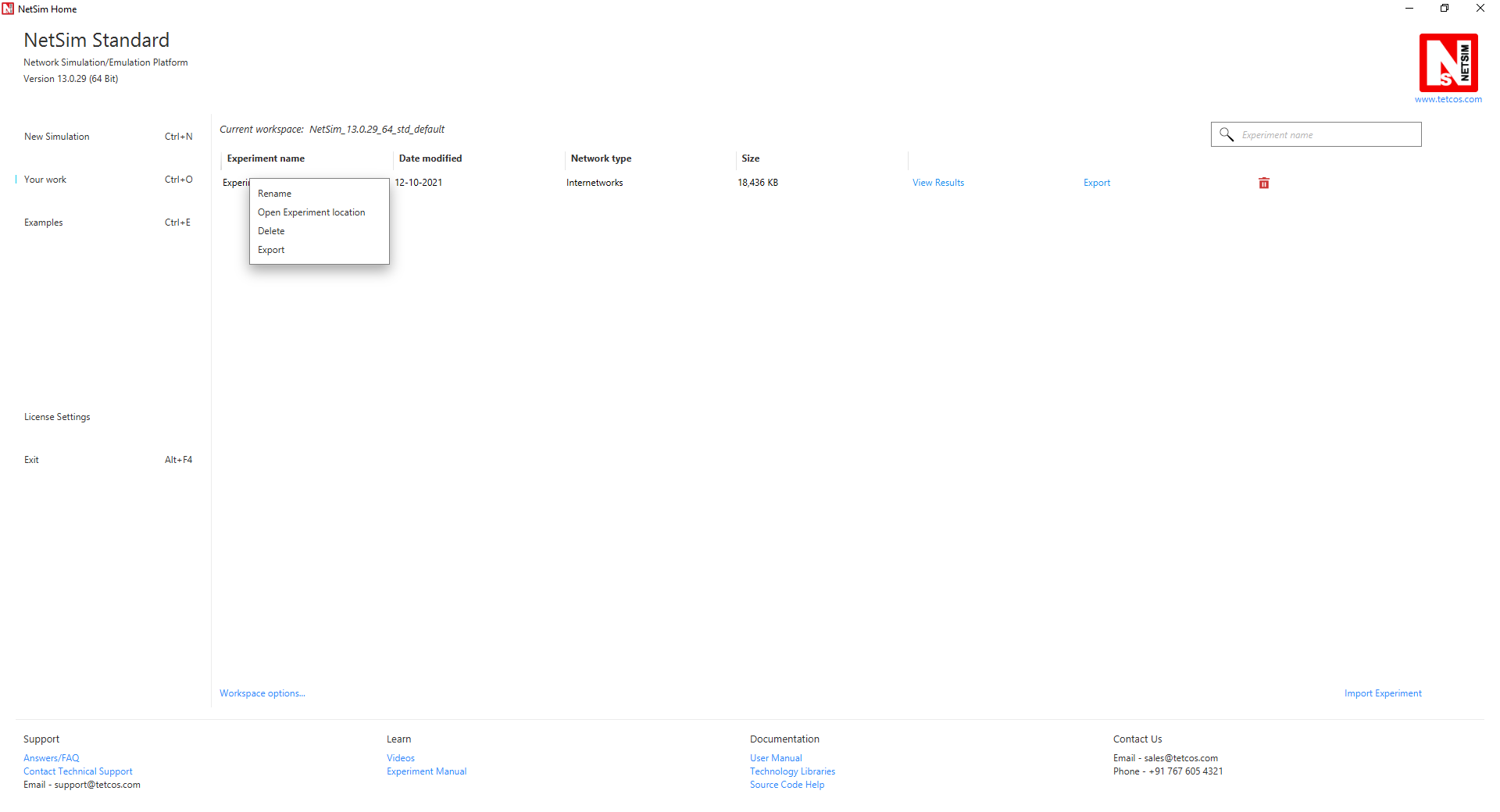NetSim plots Instantaneous (50 ms averaging window), Cumulative moving average, and Time Average for link and application throughputs. The plots can be enabled via the NetSim GUI prior to simulation.

Notes:

1. To accelerate plotting, NetSim uses down-sampling/decimation to choose n points from N for plotting.

• NetSim generates n random numbers from a discrete uniform ?? ( 0, ?? − 1) distribution and plots these n points.
• To get a more precise plot users can select the min and max values in the time series and replot.

2. The link throughput is calculated as the sum of throughputs in both directions for a full-duplex link.

3. Application throughput is plotted till the last packet reaches or end of simulation time,

whichever is earlier.

4. Cumulative Moving Average: This is the average of the metric up until the current time.

Enabling Plots in NetSim:

To enable the plots, click on the Plots option in the toolbar ribbonThis will provide plots window with the plots enabled checkbox, and clicking on ok button will enable plots for all the applications and links present in the experiment.Accessing NetSim Plots:

The Application Throughput Plots and Link Throughput Plots windows will be automatically opened after the simulation. Further plots can be accessed via Plots section in the results dashboard.Accessing raw data of NetSim Plots:

The raw data of the application throughput plots that are shown after the simulation can be easily accessed by clicking on the source data button present in the plot window as shown below:This will open the log file which contains the time stamps and the bytes transmitted as part of the application at different points of simulation time.Note: The Throughput plot log data of NetSim does not contain the X - Y values shown in the graph. This means the values are not the instantaneous/cumulative/time-average throughputs. Instead, the data consists of time stamps and bytes transmitted. From this data, the throughput (Y-axis plot value) is derived and plotted by NetSim.

The log files can also be found in the simulation saved directory if the experiment is saved after the simulation.
The saved directory of an experiment can be quickly accessed by right-clicking on the experiment name in the Open Simulation list and selecting the Open Experiment Location option as shown below:The saved directory will contain the raw data of each of the plots that were generated as part of an experiment in separate text files as shown below: